Solutions requiring more than one operationCustom SearchSOLUTIONS REQUIRING MORE THAN ONE OPERATION Most equations involve more steps in their solutions than the simple equations already described, but the basic operations remain unchanged. If the basic axioms are kept well in mind, these more complicated equations will not become too difficult. Equations may require one or all of the basic operations before a solution can be obtained. Subtraction and Division Find the value of x in the following equation:         2x + 4 = 16 1. The term containing x is isolated on the left by subtracting 4 from the left member. This operation must be balanced by also subtracting 4 from the right member, as follows:         2x + 4 - 4 = 16 - 4 2. Performing the indicated operations, we have 2x = 12 3. The multiplier 2 is removed from the x by dividing both sides of the equation by 2, as follows:Addition, Multiplication, and Division Find the value of y in the following equation: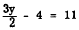1. Isolate the term containing y on the left by adding 4 to both sides, as follows: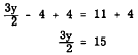2. Since the 2 will not divide the 3 exactly, multiply the left member by 2 in order to eliminate the fraction. This operation must be balanced by multiplying the right member by 2, as follows :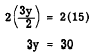3. Divide both members by 3, in order to isolate the y in the left member, as follows: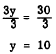Equations Having the Variable in More Than One Term Find the value of x in the following equation: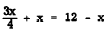1. Rewrite the equation with no terms containing the variable in the right member. This requires adding x to the right member to eliminate the -x term, and balance requires that we also add x to the left member, as follows: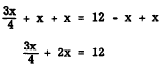2. Since the 4 will not divide the 3 exactly, it is necessary to multiply the first term by 4 to eliminate the fraction. However, notice that this multiplication cannot be performed on the first term only; any multiplier which is introduced for simplification purposes must be applied to the entire equation. Thus each term in the equation is multiplied by 4, as follows:3. Add the terms containing x and then divide both sides by 11 to isolate the x in the left member, as follows: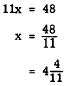Practice problems. Solve each of the following equations: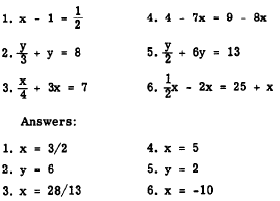Integrated Publishing, Inc. - A (SDVOSB) Service Disabled Veteran Owned Small Business